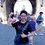# Olympiad Inequality - A not-so-Open Problem

I saw this problem online some time ago, and I have been trying to solve this inequality:

$$x,y,z >0$$, prove $\frac{x^4}{8x^3+5y^3}+\frac{y^4}{8y^3+5z^3}+\frac{z^4}{8z^3+5x^3} \geqslant \frac{x+y+z}{13}$

This question was asked online years ago and no one has proved it with an "elegant" way. I decided to share it here to all who haven't seen this problem yet, as I find it interesting and exciting while solving the problem (although I haven't found an elegant proof) XD

Have fun!

Upon typing the title I remembered a quote I saw in Evan Chen's book :D :

Graders received some elegant solutions, some not-so-elegant solutions, and some so-not-elegant solutions. --MOP 2012Note by Hua Zhi Vee
2 years, 8 months ago

This discussion board is a place to discuss our Daily Challenges and the math and science related to those challenges. Explanations are more than just a solution — they should explain the steps and thinking strategies that you used to obtain the solution. Comments should further the discussion of math and science.

When posting on Brilliant:

• Use the emojis to react to an explanation, whether you're congratulating a job well done , or just really confused .
• Ask specific questions about the challenge or the steps in somebody's explanation. Well-posed questions can add a lot to the discussion, but posting "I don't understand!" doesn't help anyone.
• Try to contribute something new to the discussion, whether it is an extension, generalization or other idea related to the challenge.

MarkdownAppears as
*italics* or _italics_ italics
**bold** or __bold__ bold
- bulleted- list
• bulleted
• list
1. numbered2. list
1. numbered
2. list
Note: you must add a full line of space before and after lists for them to show up correctly
paragraph 1paragraph 2

paragraph 1

paragraph 2

[example link](https://brilliant.org)example link
> This is a quote
This is a quote
    # I indented these lines
# 4 spaces, and now they show
# up as a code block.

print "hello world"
# I indented these lines
# 4 spaces, and now they show
# up as a code block.

print "hello world"
MathAppears as
Remember to wrap math in $$ ... $$ or $ ... $ to ensure proper formatting.
2 \times 3 $2 \times 3$
2^{34} $2^{34}$
a_{i-1} $a_{i-1}$
\frac{2}{3} $\frac{2}{3}$
\sqrt{2} $\sqrt{2}$
\sum_{i=1}^3 $\sum_{i=1}^3$
\sin \theta $\sin \theta$
\boxed{123} $\boxed{123}$

Sort by:

There is a solution that involves using symmetric polynomials, but you will need a good computer to do this.

- 2 years, 8 months ago

This problem can be solved using just pen and paper.

This inequality was used as a proposal problem for National TST of an Asian country a few years back. However, upon receiving the official solution, the committee decided to drop this problem immediately. They don't believe that any students can solve this problem in 3 hour time frame.

- 2 years, 8 months ago

Of course you can, but a proper proof would require symmetric polynomials, for which simplifying them (in this case) would be a right piece of work for a computer, let alone a human being. Fortunately, I have a very good PC that can help me out here; many others on this website would struggle to do so.

- 2 years, 8 months ago

Ok. I was wondering if it could be solved using inequalities/theorems like these.

- 2 years, 8 months ago

- 1 year, 6 months ago

Hey,

$\displaystyle \sum_{cyclic} \frac{x^4}{8x^3 + 5y^3} \geq \frac{x+y+z}{13} \geq \frac{3(xyz)^\frac{1}{3} }{13}$

So, can we prove $\displaystyle \ \ \ \sum_{cyclic} \frac{x^4}{8x^3 + 5y^3} \geq \frac{3(xyz)^\frac{1}{3} }{13}$

Cause if we can, Then it is quite easy.... IN THE CASE WHEN $\ \ x,y,z \geq 0$

- 1 year, 6 months ago

SOLUTION

$\displaystyle \sum_{cyclic} \frac{x^4}{8x^3 + 5y^3} \geq \frac{x+y+z}{13} \geq \frac{3(xyz)^\frac{1}{3} }{13}$

So We can say $\displaystyle \ \ \ \sum_{cyclic} \frac{x^4}{8x^3 + 5y^3} \times 13(x^3 + y^3 +z^3) \geq (x^2 + y^2 + z^2)^2 \ \ \ \$ By Cauchy-Schwarz Inequality more precisely Titu's Lemma

Also we can see that $\displaystyle \ \ x^2 + y^2 + z^2 \geq xy + yz + zx$

Thus, $\displaystyle \ \sum_{cyclic} \frac{x^4}{8x^3 + 5y^3} \times (x^3 + y^3 +z^3) \geq \frac {(xy + yz + zx)^2}{13}$

And, $\displaystyle \ \ \frac {(xy + yz + zx)^2}{13} \geq \frac {9(xyz)^ \frac {4}{3} }{13} \ \$ By AM-GM-HM Inequality

Finally, $\displaystyle \ \ \frac {(xy + yz + zx)^2}{13} \geq \frac {3(xyz)^ \frac {1}{3} }{13} \times 3xyz \ \$

So$\displaystyle \ \sum_{cyclic} \frac{x^4}{8x^3 + 5y^3} \times (x^3 + y^3 +z^3) \geq \frac {3(xyz)^ \frac {1}{3} }{13} \times 3xyz$........................1

Also, $\displaystyle \ \ x^3 + y^3 +z^3 \geq 3xyz$.....................2

Now, as $\displaystyle \ \ x,y,z \geq 0 \ \$ So divide inequality 1 by inequality 2 ,

Hence the final inequality becomes $\displaystyle \ \ \ \sum_{cyclic} \frac{x^4}{8x^3 + 5y^3} \geq \frac{3(xyz)^\frac{1}{3} }{13}$

If it wasn't metioned that$\displaystyle \ \ x,y,z \geq 0 \ \$ we couldn't divide two inequalities directly.

- 1 year, 6 months ago

Yes, you did prove that $\sum_{cyc} \frac{x^4}{8x^3+5y^3} \geq \frac{3(xyz)^{\frac13}}{13}$, but unfortunately, that doesn't quite prove the original problem statement. This is because $\sum_{cyc} \frac{x^4}{8x^3+5y^3} \geq \frac{3(xyz)^{\frac13}}{13}$ can definitely mean $\frac{x+y+z}{13} \geq \sum_{cyc} \frac{x^4}{8x^3+5y^3} \geq \frac{3(xyz)^{\frac13}}{13}$.

I'm gonna give a simple example. Suppose that you want to prove $x > y$. You DO know that $y>z$. And somehow you do manage to prove that $x>z$. Does it mean that $x>y$?

No! The conditions $y>z$ and $x>z$ does not imply that $x>y$. See: If $x=2,y=3,z=1$: $y=3>z=1$ TRUE, and $x=2>z=1$ TRUE, however, $x=2!

- 1 year, 4 months ago

Yes, I agree with you.. Thank you for correcting me.

- 1 year, 4 months ago

There, if you're interested in more inequalities :) https://artofproblemsolving.com/articles/files/MildorfInequalities.pdf

- 1 year, 4 months ago

I will surely access it.

- 1 year, 4 months ago